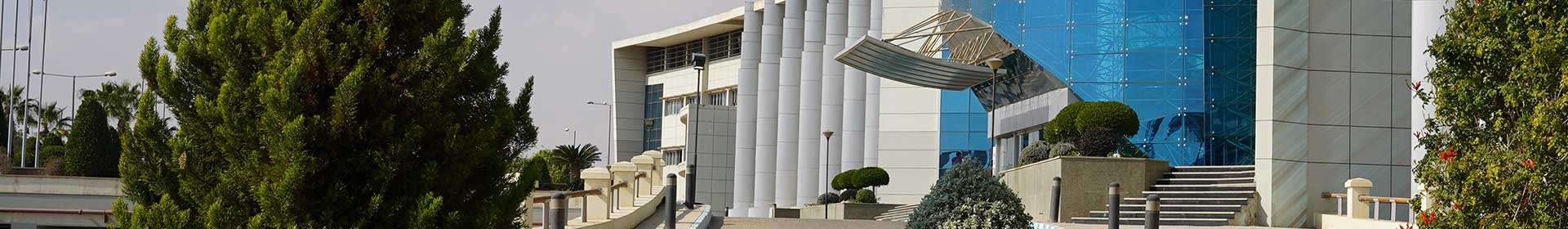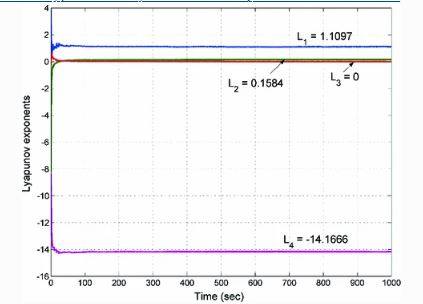Journal

# Hyperchaos and adaptive control of a novel hyperchaotic system with two quadratic nonlinearities

By
Vaidyanathan S.
Azar A.T.
Ouannas A.

Liu-Su-Liu chaotic system (2007) is one of the classical 3-D chaotic systems in the literature. By introducing a feedback control to the Liu-Su-Liu chaotic system,we obtain a novel hyperchaotic system in this work, which has two quadratic nonlinearities. The phase portraits of the novel hyperchaotic system are displayed and the qualitative properties of the novel hyperchaotic system are discussed. We show that the novel hyperchaotic system has a unique equilibrium point at the origin, which is unstable. The Lyapunov exponents of the novel 4-D hyperchaotic system are obtained as L1 = 1.1097, L2 = 0.1584, L3 = 0 and L4 = −14.1666. The maximal Lyapunov exponent (MLE) of the novel hyperchaotic system is obtained as L1 = 1.1097 and Lyapunov dimension as DL = 3.0895. Since the sum of the Lyapunov exponents of the novel hyperchaotic system is negative, it follows that the novel hyperchaotic system is dissipative. Next, we derive new results for the adaptive control design of the novel hyperchaotic system with unknown parameters. We also derive new results for the adaptive synchronization design of identical novel hyperchaotic systems with unknown parameters. The adaptive control results derived in this work for the novel hyperchaotic system are proved using Lyapunov stability theory. Numerical simulations in MATLAB are shown to validate and illustrate all the main results derived in this work. © Springer International Publishing AG 2017.